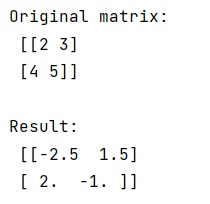# How to inverse a matrix using NumPy?

Learn, how to inverse a matrix using NumPy in Python?
Submitted by Pranit Sharma, on January 15, 2023

NumPy is an abbreviated form of Numerical Python. It is used for different types of scientific operations in python. Numpy is a vast library in python which is used for almost every kind of scientific or mathematical operation. It is itself an array which is a collection of various methods and functions for processing the arrays.

## Inversing a matrix using NumPy

Mathematically,

The inverse of matrix is another matrix, which on multiplication with the given matrix gives the multiplicative identity. For a matrix A, its inverse is A-1, and A · A-1 = A-1· A = I, where I is the identity matrix. The matrix whose determinant is non-zero and for which the inverse matrix can be calculated is called an invertible matrix.

Suppose, we are given with a numpy matrix and we need to create another matrix which is its inverse.

For this purpose, we will first create a numpy matrix and then we will use the I attribute which is used to generate an inverse of that matrix along which it is used.

Note: The I attribute only works with matrix.

Let us understand with the help of an example,

## Python code to inverse a matrix using NumPy

```# Import numpy
import numpy as np

# Import pandas
import pandas as pd

# Creating a numpy matrix
mat = np.matrix([[2,3],[4,5]])

# Display original matrix
print("Original matrix:\n",mat,"\n")

# Finding matrix inverse
res = mat.I

# Display result
print("Result:\n",res)
```

Output:What's New (MCQs)

Top Interview Coding Problems/Challenges!

IncludeHelp's Blogs

Languages: » C » C++ » C++ STL » Java » Data Structure » C#.Net » Android » Kotlin » SQL
Web Technologies: » PHP » Python » JavaScript » CSS » Ajax » Node.js » Web programming/HTML
Solved programs: » C » C++ » DS » Java » C#
Aptitude que. & ans.: » C » C++ » Java » DBMS
Interview que. & ans.: » C » Embedded C » Java » SEO » HR
CS Subjects: » CS Basics » O.S. » Networks » DBMS » Embedded Systems » Cloud Computing
» Machine learning » CS Organizations » Linux » DOS
More: » Articles » Puzzles » News/Updates